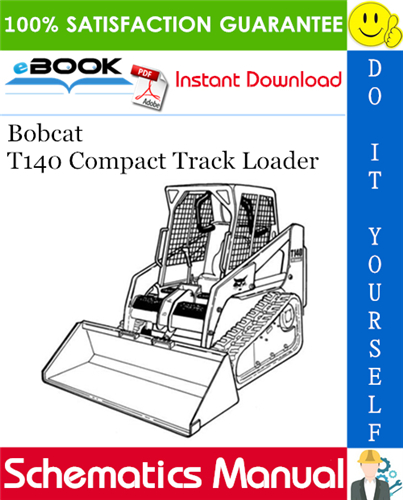Complete WIRING/HYDRAULIC/HYDROSTATIC SCHEMATIC for the Bobcat T140 Compact Track Loader.

This manual contains high quality images, Schematics and instructions to help you to operate and repair your Machine.

Covers the following serial numbers:
=========
T140 (S/N A3L720001 AND ABOVE)
T140 (S/N A3L820001 AND ABOVE)
T140 (S/N A3L711001 – A3L719999)
T140 (S/N A3L811001 – A3L819999)
T140 (S/N A3L711001 – A3L719999)
T140 (S/N A3L811001 – A3L819999)
T140 (S/N 529311001 AND ABOVE)
T140 (S/N 531311001 AND ABOVE)
T140 (S/N 529311882 AND ABOVE)
T140 (S/N A8M511003 AND ABOVE)
T140 (S/N 531311582 AND ABOVE)
T140 (S/N 529311592 AND ABOVE)
T140 (S/N A8M511001 AND ABOVE)
T140 (S/N 531311264 – 531311581)
T140 (S/N 531311264 AND ABOVE)
T140 (S/N 529311001 – 529311881)
T140 (S/N A8M511001 – A8M511002)
T140 (S/N 529311001 – 529311591)
T140 (S/N 531311001 – 531311263)
T140 (S/N 527111001 AND ABOVE)
T140 (S/N 527211001 AND ABOVE)

=========

Model Specification: Bobcat T140 Compact Track Loader
Language: English
File Format: PDF